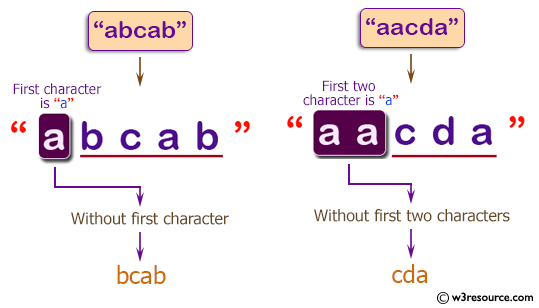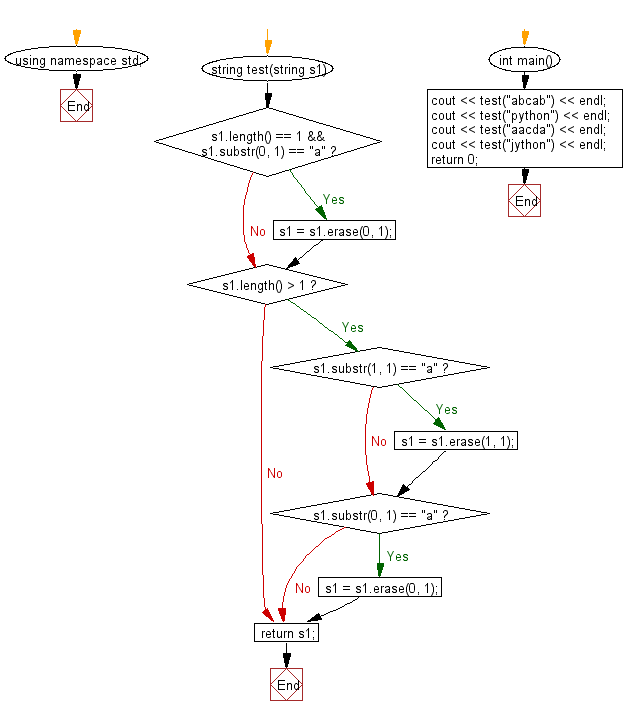﻿ C++ : String without the first or first 2 characters# C++ Exercises: Create a new string from a given string. If the first or first two characters is 'a', return the string without those 'a' characters otherwise return the original given string

## C++ Basic Algorithm: Exercise-80 with Solution

Write a C++ program to create a new string from a given string. If the first or first two characters is 'a', return the string without those 'a' characters otherwise return the original given string.

Sample Solution:

C++ Code :

``````#include <iostream>
using namespace std;

string test(string s1)
{
if (s1.length() == 1 && s1.substr(0, 1) == "a")
{
s1 = s1.erase(0, 1);
}

if (s1.length() > 1)
{
if (s1.substr(1, 1) == "a")
{
s1 = s1.erase(1, 1);
}

if (s1.substr(0, 1) == "a")
{
s1 = s1.erase(0, 1);
}
}

return s1;
}

int main()
{
cout << test("abcab") << endl;
cout << test("python") << endl;
cout << test("aacda") << endl;
cout << test("jython") << endl;
return 0;
}
``````

Sample Output:

```bcab
python
cda
jython
```

Pictorial Presentation:Flowchart:C++ Code Editor:

Contribute your code and comments through Disqus.

What is the difficulty level of this exercise?

﻿